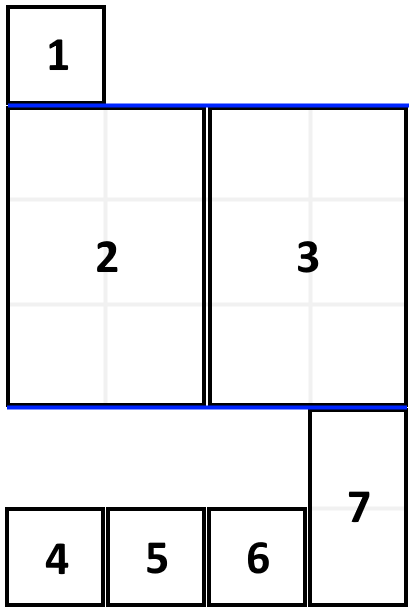# 填充书架

## 1105. 填充书架 (Medium)```输入：books = [[1,1],[2,3],[2,3],[1,1],[1,1],[1,1],[1,2]], shelf_width = 4

3 层书架的高度和为 1 + 3 + 2 = 6 。

```

• `1 <= books.length <= 1000`
• `1 <= books[i] <= shelf_width <= 1000`
• `1 <= books[i] <= 1000`

[数组] [动态规划]